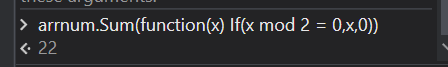# Array Manipulation (Sum of the only Even number)

Array Manipulation (Sum of the only Even number)

Input : 2, 4, 6, 8, 10
Output: Sum of even numbers = 30

Input : 1, 3, 5, 7, 9
output: there is no even numbers

Input: 0, 1, 2, 3, 4, 5, 6, 7
Output: Sum of even numbers = 12

Hi

U can try with this expression in a assign activity

Let’s say u have this input numbers in a array variable named arr

Then in assign activity

str_output =
arr.Where(Function(x) x Mod 2 = 0).Sum().ToString

Check this out for more details

Hope this helps

Cheers @shaik.muktharvalli1

@shaik.muktharvalli1

Try this

`arrayVariable.Sum(function(x) If(x mod 2 = 0,x,0))`

this gives sum as 0 when no even number is present …else sum would be a valid numbercheers

Hello @shaik.muktharvalli1

Dim numbersArray As Integer() = {2, 4, 6, 8, 10}
Dim evenSum As Integer = 0
Dim evenFound As Boolean = False

For Each number In numbersArray
If number Mod 2 = 0 Then
evenSum += number
evenFound = True
End If
Next

If evenFound Then
MessageBox.Show("Sum of even numbers = " & evenSum.ToString())
Else
MessageBox.Show(“There are no even numbers”)
End If

Thanks & Cheers!!!

Hi,

you can use this query

Input.Sum(Function(x) If(x Mod 2 = 0, x, 0))